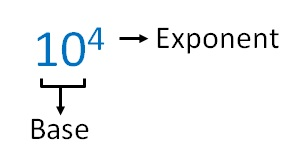Exponents

Chapter 11 Class 7 Exponents and Powers
Concept wiseWhat does 10 4 mean?

It means 10 multiplied 4 times

10 4 = 10 × 10 × 10 × 10

Similarly

5 2 = 5 × 5

2 3 = 2 × 2 × 2

4 8 = 4 × 4 × 4 × 4 × 4 × 4 × 4 × 4

3 5 = 3 × 3 × 3 × 3 × 3

Here,

10 4 is called 10 to the power of 4

5 2 is called 5 to the power of 2 or 5 squared

2 3 is called 2 to the power of 3 or 2 cubed

4 8 is called 4 to the power of 8

3 5 is called 3 to the power of 5

Learn in your speed, with individual attention - Teachoo Maths 1-on-1 Class